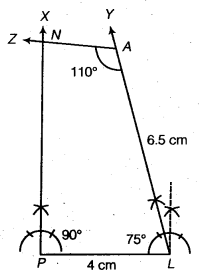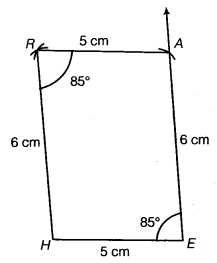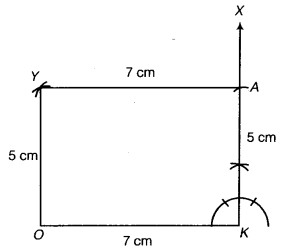# Class 8 Maths NCERT Solutions for Chapter – 4 Practical Geometry Ex – 4.3

## Practical Geometry

Question 1.

MO = 6 cm
OR = 4.5 cm
∠M = 60°
∠O = 105°
∠R = 105°

PL = 4 cm
LA = 6.5 cm
∠P = 90°
∠A = 110°
∠N – 85°.

(iii) Parallelogram HEAR
HE = 5 cm
EA = 6 cm
∠R = 85°

(iv) Rectangle OKAY
OK = 7 cm
KA = 5 cm.

Solution.
(i) Draw a rough sketch of the required quadrilateral and write down its dimensions.

Steps of Construction :

• Draw MO = 6 cm.
• Make ∠MOX = 105°.
• With O as centre and radius 4.5 cm, cut off OR = 4.5 cm along OX.
• Make ∠ORY = 105°.
• Make ∠ZMO = 60° such that arms RY and MZ intersect at E.
Then, MORE is the required quadrilateral.

(ii) Draw a rough sketch of the required quadrilateral PLAN and write down its dimensions.
Before taking up the construction let us find the ∠PLA which is equal to 360° – (90°+ 110°+ 85°)
= 360°-285° = 75°.

Steps of Construction :• Draw PL = 4 cm.
• Make ∠XPL =90°.
• Make ∠YLP = 75°.
• With L as centre and radius 6.5 cm, cut off LA – 6.5 cm along LY.
• Make ∠ZAL =110° and let its arm AZ intersect PX at N.
Then, PLAN is the required quadrilateral.

(iii) We know that the opposite angles of a parallelogram are equal. Make a rough sketch of the required parallelogram as shown.

Steps of Construction :• Draw HE = 5 cm.
• Make ∠HEX = 85°.
• With E as centre and radius 6 cm, cut off EA = 6 cm along EX.
• With A as centre and radius 5 cm, draw an arc.
• With H as centre and radius 6 cm, draw another arc cutting the previous arc at R.
• Join HR and AR.
Then, HEAR is the required parallelogram.

(iv) We known that the opposite sides of a rectangle are equal and each angle of it is 90°.
Make a rough sketch of the required
rectangle and write down its dimensions.

Steps of Construction :• Draw OK = 7 cm.
• Draw ∠OKX = 90°.
• With K as centre and radius 5 cm, draw an arc KX at A.
• With A as centre and radius 7 cm, draw an arc.
• With O as centre and radius 5 cm, draw another arc cutting the previous arc at Y.
• Join AY and OY.
Then, OKAY is the required rectangle.

### Take Your IIT JEE Coaching to Next Level with Examtube

• Mentoring & Teaching by IITians
• Regular Testing & Analysis
• Preparation for Various Engineering Entrance Exams
• Support for School/Board Exams